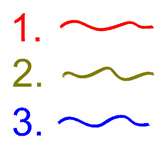Definition of

# AlgorithmA step-by-step solution.

Each step has clear instructions. Like a recipe.

Example: one algorithm for adding two digit numbers is:
1. add the tens
2. add the ones
3. add the numbers from steps 1 and 2

So to add 15 and 32 using that algorithm:
1. add 10 and 30 to get 40
2. add 5 and 2 to get 7
3. add 40 and 7 to get 47

Long Division is another example of an algorithm: when you follow the steps you get the answer.

Computers use algorithms all the time.

"Algorithm" is named after the 9th century Persian mathematician Al-Khwarizmi.